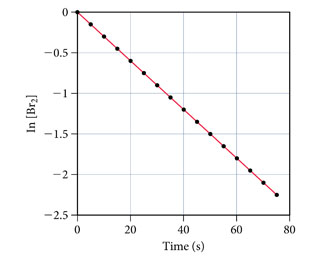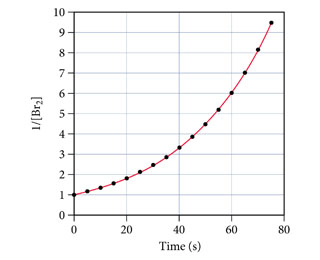# Problem: The decomposition of Br2 is followed as a function of time; two different plots of the data are shown here. Determine the order and rate constant for the reaction.

###### FREE Expert Solution

plot with straight line  order for the reaction

plot of ln[Br2] vs time  straight line

83% (411 ratings)###### Problem Details

The decomposition of Br2 is followed as a function of time; two different plots of the data are shown here.Determine the order and rate constant for the reaction.